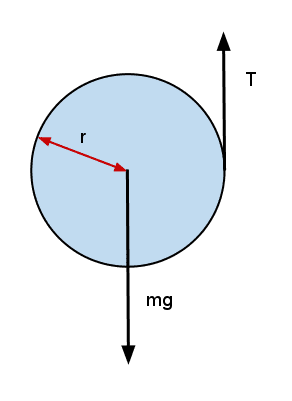# Force exerted by a rope wound around a disk

• I
• Mukhtar Jafri
In summary, the conversation discusses the forces acting on a disk supported by a rope wound around it. The tension T is shown to be acting where the rope loses contact with the disk, due to the assumed high friction. However, there is also a reaction force from the rope, which may not cancel out completely due to the weight of the disk. The question of how to account for all these forces is raised, and it is suggested to consider the rope and disk as one system, resulting in internal forces. The remaining external tension force is shown to act along the line of the rope. A response is given, acknowledging the difficulty of accounting for these forces and suggesting an alternative approach.

#### Mukhtar JafriThe above picture shows the forces on a disk supported by a rope which is wound around it. The tension T is shown to be acting at the point where the rope loses contact with the disk. I think the reason for this is that the friction is assumed to be huge so that the tension in the rope also acts on the disk. But there is also the reaction from the rope and since the rope is wound around the entire circumference we may think that it cancels out but I think that the reaction from the rope at the bottom should be greater than at the top due to the weight of the disk. How to account for all these forces? And if it is true that the net force by the rope on the disk is just the tension T as shown then how can I prove it precisely?

#### Attachments

Mukhtar Jafri said:
How to account for all these forces?
Accounting for those forces would indeed be very difficult. So I would take a different approach. Instead of considering the rope and the disk to be separate systems, I would consider them to be part of the same system. Then all of those forces between the disk and the rope become internal forces. The remaining external tension force is clearly acting along the line of the rope as shown.

•jrmichler and 256bits
Dale gave a really good answer.

Dale said:
Accounting for those forces would indeed be very difficult. So I would take a different approach. Instead of considering the rope and the disk to be separate systems, I would consider them to be part of the same system. Then all of those forces between the disk and the rope become internal forces. The remaining external tension force is clearly acting along the line of the rope as shown.
Thank you very much. I completely missed that.

## 1. What is the purpose of winding a rope around a disk?

The purpose of winding a rope around a disk is to increase the amount of force that can be exerted on an object. This is because when a rope is wound around a disk, it creates a pulley system, which allows for the force to be distributed over a larger area.

## 2. How does the number of times the rope is wound around the disk affect the force exerted?

The number of times the rope is wound around the disk directly affects the force that can be exerted. The more times the rope is wound, the greater the force that can be exerted due to the increased surface area and leverage provided by the pulley system.

## 3. Is the force exerted by the rope on the disk equal to the force exerted by the disk on the rope?

According to Newton's third law of motion, the force exerted by the rope on the disk is equal in magnitude and opposite in direction to the force exerted by the disk on the rope. This is because the rope and disk are in contact with each other and interact with equal and opposite forces.

## 4. Can the force exerted by a rope wound around a disk be increased indefinitely?

No, the force exerted by a rope wound around a disk cannot be increased indefinitely. This is because there are limits to the amount of tension a rope can withstand before breaking, and there are limits to the strength and stability of the disk itself.

## 5. How does friction affect the force exerted by a rope wound around a disk?

Friction can affect the force exerted by a rope wound around a disk by reducing the efficiency of the pulley system. This is because friction creates resistance and can cause the rope to slip or become stuck, which decreases the amount of force that can be exerted.